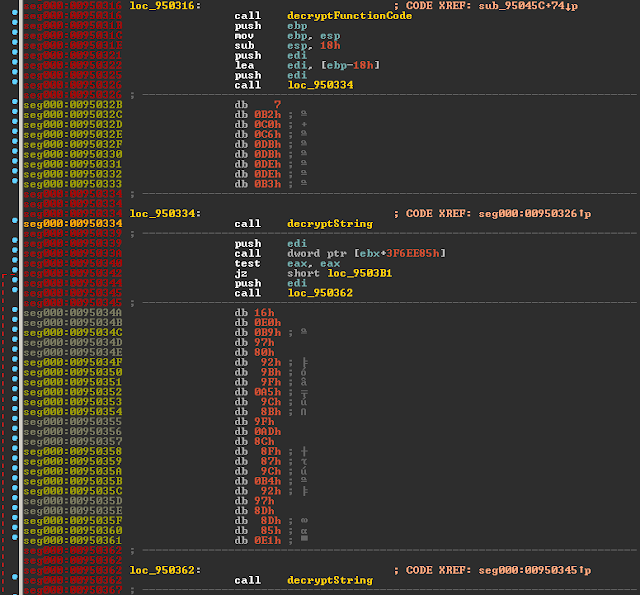## 18.08.2015

### Knowledge Fragment: Fobber Inline String Decryption

In the other blog post on Fobber, I have demonstrated how to batch decrypt function code, which left us with IDA recognizing a fair amount of code opposed to only a handful of functions:After function decryption, IDA recognizes some code already.
However, we can see that there is still a lot of "red" remaining, meaning that functions have not been recognized as nicely as we would like it.

The reason for this is that Fobber uses another technique which we might call "inline string decryption".
It looks like this:Fobber inline calls at 0x950326 and 0x950345, pushing the crypted string address to the stack which is then consumed as argument by decryptString()
We can see two calls to decryptString(), and both of them are preceded by a collection of bytes prior to which a call happens that seemingly "jumps" over them.
The effect of a call is that it pushes its return address to the stack - in our case resulting in the absolute address of the encrypted string directly following this call being pushed to the stack. From a coder's perspective, this "calling over the encrypted string" is an elegant way to save a couple bytes, while from an analysts perspective, this really screws with IDA. :)

Let's look at how the strings are decrypted:Fobber's string decryption function.
Again, the rather simple single-byte xor-loop jumps the eye.

However, the interesting part is how parameters are loaded.

Thus, let me explain the effects of instructions one-by-one:

[...]
mov   edi, [ebp+0Ch]       | move pointer where decrypted string will be put to EDI
mov   esi, [ebp+8]         | move pointer of encrypted string to ESI
lodsw                      | load two bytes (word) from ESI
movzx ecx, al              | put the lower byte into ecx and zero extend -> this is our len
lodsb                      | load another byte from ESI (first byte of encrypted string)
xor   al, ah               | xor string byte with upper byte loaded by lodsw (our key)
xor   al, cl               | xor string byte with number of remaining chars (CL)
stosb                      | store decrypted byte
loop  loc_953878           | decrement ECX and repeat as long as >0.
[...]

Running this as exmaple for the first encrypted string as shown in the first picture:

crypted string len
|  key
|  |
remaining len           |  |  07 06 05 04 03 02 01
07 B2 C0 C6 DB DB DE DE B3
xor key (B2)            -- -- 72 74 69 69 6c 6c 01
xor remaining len       -- -- 75 72 6c 6d 6f 6e 00
ASCII                   -- --  u  r  l  m  o  n --

So the first decrypted string here resolves nicely to "urlmon".
Let's automate for all strings again.

### Decrypt All The Strings

First, we locate the string decryption function.
This time we can use regex r"\xE8....\x55\x89\xe5\x60.{8,16}\x30.\x30" which again gives a unique hit.

This time, we first locate all calls to this function, like in the post on function decryption. For this we can use the regex r"\xE8" again to find all potential "call rel_offset" instructions.
We apply the same address math and check if the call destination (calculated as: image_base + call_origin + relative_call_offset + 5) is equal to the address of our string decryption function.
In this case, we can store the call_origin as a candidate for string decryption.

Next, we run again over all calls and check if a call to one of these string decryption candidates happens - this is very likely one of the "calling over encrypted strings" locations as explained earlier. This could probably have been solved differently but it worked for me.
Next we extract and decrypt the string, then patch it again in the binary.
I also change the "call over encrypted string" to a jump (first byte 0xE8->0xE9) because IDA likes this more and will not create wrongly detected functions later on.

Code:

#!/usr/bin/env python
import re
import struct

def decrypt_string(crypted_string):
decrypted = ""
size = ord(crypted_string)
key = ord(crypted_string)
remaining_chars = len(crypted_string[2:])
index = 0
while remaining_chars > 0:
decrypted += chr(ord(crypted_string[2 + index]) ^ remaining_chars ^ key)
remaining_chars -= 1
index += 1
return decrypted + "\x00\x00"

def replace_bytes(buf, offset, bytes):
return buf[:offset] + bytes + buf[offset + len(bytes):]

def decrypt_all_strings(binary, image_base):
# locate decryption function
decrypt_string_offset = re.search(r"\xE8....\x55\x89\xe5\x60.{8,16}\x30.\x30", binary).start()

# locate calls to decryption function
regex_call = r"\xe8"
calls_to_decrypt_string = []
for match in re.finditer(regex_call, binary):
call_origin = match.start()
packed_call = binary[call_origin + 1:call_origin + 1 + 4]
rel_call = struct.unpack("I", packed_call)
call_destination = (image_base + call_origin + rel_call + 5) & 0xFFFFFFFF
if call_destination == image_base + decrypt_string_offset:
calls_to_decrypt_string.append(image_base + call_origin)

# identify calls to these string decryption candidates
for match in re.finditer(regex_call, binary):
call_origin = match.start()
packed_call = binary[call_origin + 1:call_origin + 1 + 4]
rel_call = struct.unpack("I", packed_call)
call_destination = (image_base + call_origin + rel_call + 5) & 0xFFFFFFFF

if call_destination in calls_to_decrypt_string:

# decrypt string and fix in the binary
crypted_string = binary[call_origin + 0x5:call_destination -  image_base]
decrypted_string = decrypt_string(crypted_string)
binary = replace_bytes(binary, call_origin, "\xE9")
binary = replace_bytes(binary, call_origin + 0x5, decrypted_string)
print "0x%x: %s" % (image_base + call_origin, decrypted_string)

return binary

[...]

Load in IDA and see the result:Decryption of all "call over encrypted string" locations.
Yay.

### Conclusion

This blog post detailed how Fobber uses encrypted inline strings, which sadly is also a big deal to IDA, misclassifying a bunch of calls.

sample used:
md5: 49974f869f8f5d32620685bc1818c957
1.